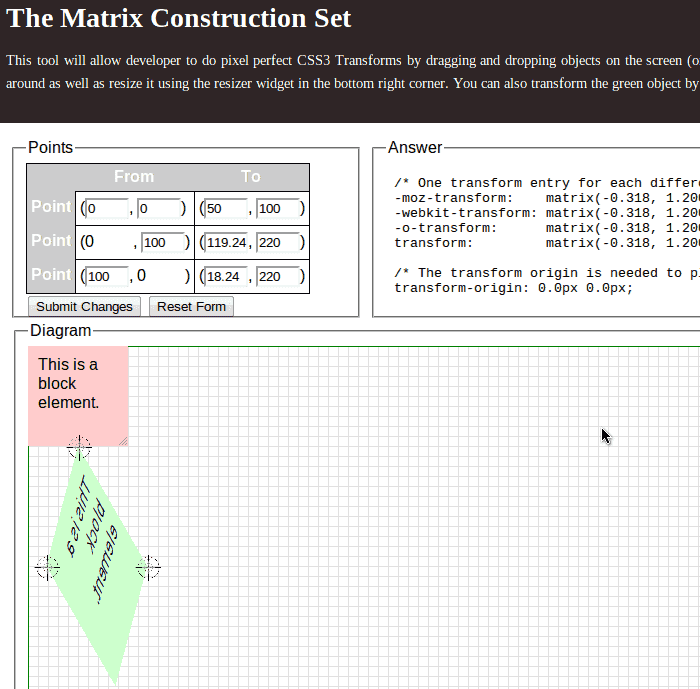matrix[1, 2, 3, 4, 5, 6] =
1 3 5
2 4 6
0 0 1

scale(a) =
a 0 0
0 a 0
0 0 1
scaleX(x) =
x 0 0
0 1 0
0 0 1
scaleY(y) =
1 0 0
0 y 0
0 0 1
translate(x,y) =
0 0 x
0 0 y
0 0 1
translateX(x) =
0 0 x
0 0 0
0 0 1
translateY(y) =
0 0 0
0 0 y
0 0 1
skew(x,y) =
1    tanx    0
tany   1       0
0       0      1
skewX(x) =
1  tanx  0
0     1     0
0     0     1
skewY(y) =
1     0  0
tany  1  0
0     0  1
rotate(θ) =
cosθ   -sinθ   0
sinθ    cosθ   0
0          0      1

• 1, 4 是縮放變形的結果
• 5, 6 是位移變形的結果
• 2, 3 是歪斜變形的結果
• 1, 2, 3, 4 是旋轉變形的結果

-moz-transform: matrix(0.6924, 1.2, -0.3216, 1.2, 50px, 100px);

-webkit-transform: matrix(0.6924, 1.2, -0.3216, 1.2, 50, 100);

-o-transform: matrix(0.6924, 1.2, -0.3216, 1.2, 50, 100);

transform: matrix(0.6924, 1.2, -0.3216, 1.2, 50, 100);

matrix =
0.6924  -0.3216  50
1.2        1.2     100
0           0        1

A(0,0) =
0
0
1
，B(100,0) =
100
0
1
，C(0,100) =
0
100
1
，D(100,100) =
100
100
1

A' =
50
100
1
，B' =
119.24
220
1
，C' =
17.84
220
1
，D' =
87.08
340
1

A' (50, 100)

B' (119.24, 220)
C' (18.84, 220)
D' (87.08, 340)Matrix = translate x rotate x scale x skew

-webkit-transform: translate(50px, 100px) rotate(30deg) scale(1.2) skew(15deg, 30deg);

-webkit-transform: matrix(0.6924, 1.2, -0.3216, 1.2, 50, 100);